11 Angular Momentum

# 11.3 Conservation of Angular Momentum

### Learning Objectives

By the end of this section, you will be able to:

• Apply conservation of angular momentum to determine the angular velocity of a rotating system in which the moment of inertia is changing
• Explain how the rotational kinetic energy changes when a system undergoes changes in both moment of inertia and angular velocity

So far, we have looked at the angular momentum of systems consisting of point particles and rigid bodies. We have also analyzed the torques involved, using the expression that relates the external net torque to the change in angular momentum, Figure. Examples of systems that obey this equation include a freely spinning bicycle tire that slows over time due to torque arising from friction, or the slowing of Earth’s rotation over millions of years due to frictional forces exerted on tidal deformations.

However, suppose there is no net external torque on the system, $\sum \mathbf{\overset{\to }{\tau }}=0.$ In this case, Figure becomes the law of conservation of angular momentum.

#### Law of Conservation of Angular Momentum

The angular momentum of a system of particles around a point in a fixed inertial reference frame is conserved if there is no net external torque around that point:

$\frac{d\mathbf{\overset{\to }{L}}}{dt}=0$

or

$\mathbf{\overset{\to }{L}}={\mathbf{\overset{\to }{l}}}_{1}+{\mathbf{\overset{\to }{l}}}_{2}\,\text{+}\,\cdots\,\text{+}\,{\mathbf{\overset{\to }{l}}}_{N}=\text{constant}\text{.}$

Note that the total angular momentum $\mathbf{\overset{\to }{L}}$ is conserved. Any of the individual angular momenta can change as long as their sum remains constant. This law is analogous to linear momentum being conserved when the external force on a system is zero.

As an example of conservation of angular momentum, Figure shows an ice skater executing a spin. The net torque on her is very close to zero because there is relatively little friction between her skates and the ice. Also, the friction is exerted very close to the pivot point. Both $|\mathbf{\overset{\to }{F}}|\,\text{and}\,|\mathbf{\overset{\to }{r}}|$ are small, so $|\mathbf{\overset{\to }{\tau }}|$ is negligible. Consequently, she can spin for quite some time. She can also increase her rate of spin by pulling her arms and legs in. Why does pulling her arms and legs in increase her rate of spin? The answer is that her angular momentum is constant, so that

${L}^{\prime }=L$

or

${I}^{\prime }{\omega }^{\prime }=I\omega ,$

where the primed quantities refer to conditions after she has pulled in her arms and reduced her moment of inertia. Because ${I}^{\prime }$ is smaller, the angular velocity ${\omega }^{\prime }$ must increase to keep the angular momentum constant.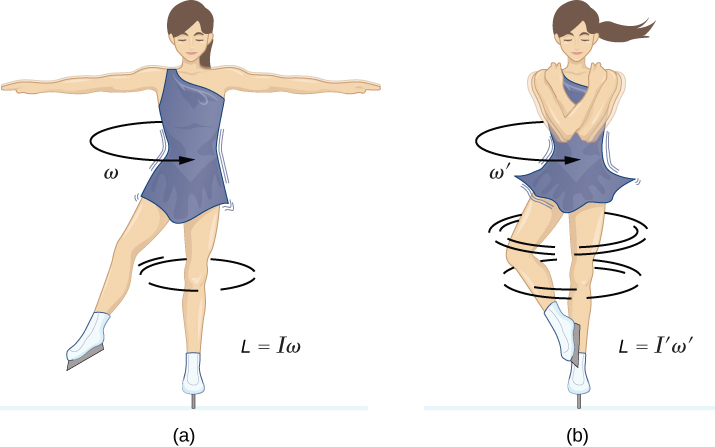Figure 11.14 (a) An ice skater is spinning on the tip of her skate with her arms extended. Her angular momentum is conserved because the net torque on her is negligibly small. (b) Her rate of spin increases greatly when she pulls in her arms, decreasing her moment of inertia. The work she does to pull in her arms results in an increase in rotational kinetic energy.

It is interesting to see how the rotational kinetic energy of the skater changes when she pulls her arms in. Her initial rotational energy is

${K}_{\text{Rot}}=\frac{1}{2}I{\omega }^{2},$

whereas her final rotational energy is

${{K}^{\prime }}_{\text{Rot}}=\frac{1}{2}{I}^{\prime }{({\omega }^{\prime })}^{2}.$

Since ${I}^{\prime }{\omega }^{\prime }=I\omega ,$ we can substitute for ${\omega }^{\prime }$ and find

${{K}^{\prime }}_{\text{Rot}}=\frac{1}{2}{I}^{\prime }{({\omega }^{\prime })}^{2}=\frac{1}{2}{I}^{\prime }{(\frac{I}{{I}^{\prime }}\omega )}^{2}=\frac{1}{2}I{\omega }^{2}(\frac{I}{{I}^{\prime }})={K}_{\text{Rot}}(\frac{I}{{I}^{\prime }})\text{​}.$

Because her moment of inertia has decreased, ${I}^{\prime } \lt I,$ her final rotational kinetic energy has increased. The source of this additional rotational kinetic energy is the work required to pull her arms inward. Note that the skater’s arms do not move in a perfect circle—they spiral inward. This work causes an increase in the rotational kinetic energy, while her angular momentum remains constant. Since she is in a frictionless environment, no energy escapes the system. Thus, if she were to extend her arms to their original positions, she would rotate at her original angular velocity and her kinetic energy would return to its original value.

The solar system is another example of how conservation of angular momentum works in our universe. Our solar system was born from a huge cloud of gas and dust that initially had rotational energy. Gravitational forces caused the cloud to contract, and the rotation rate increased as a result of conservation of angular momentum (Figure).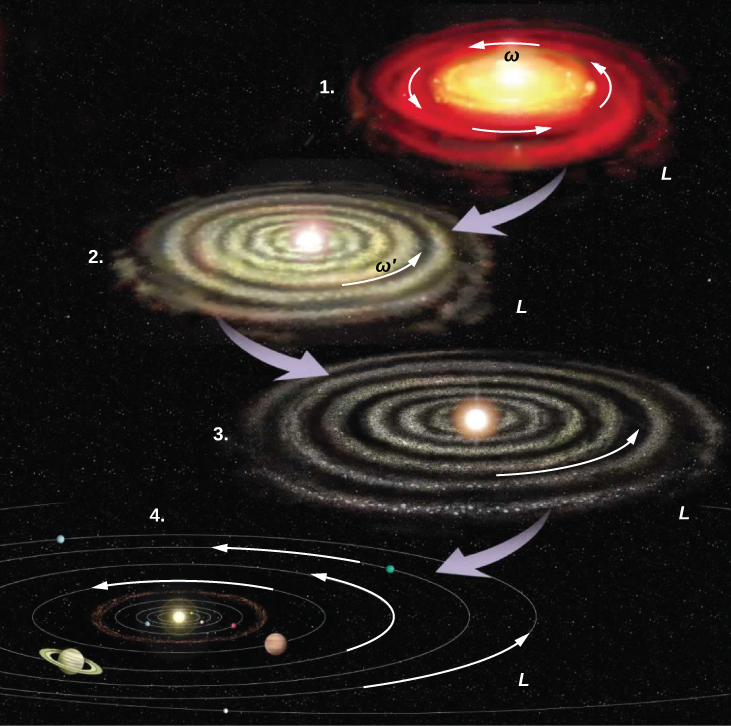Figure 11.15 The solar system coalesced from a cloud of gas and dust that was originally rotating. The orbital motions and spins of the planets are in the same direction as the original spin and conserve the angular momentum of the parent cloud. (credit: modification of work by NASA)

We continue our discussion with an example that has applications to engineering.

### Example

#### Coupled Flywheels

A flywheel rotates without friction at an angular velocity ${\omega }_{0}=600\,\text{rev}\text{/}\text{min}$ on a frictionless, vertical shaft of negligible rotational inertia. A second flywheel, which is at rest and has a moment of inertia three times that of the rotating flywheel, is dropped onto it (Figure). Because friction exists between the surfaces, the flywheels very quickly reach the same rotational velocity, after which they spin together. (a) Use the law of conservation of angular momentum to determine the angular velocity $\omega$ of the combination. (b) What fraction of the initial kinetic energy is lost in the coupling of the flywheels?

#### Strategy

Part (a) is straightforward to solve for the angular velocity of the coupled system. We use the result of (a) to compare the initial and final kinetic energies of the system in part (b).

#### Solution

a. No external torques act on the system. The force due to friction produces an internal torque, which does not affect the angular momentum of the system. Therefore conservation of angular momentum gives

$\begin{array}{c}{I}_{0}{\omega }_{0}=({I}_{0}+3{I}_{0})\omega ,\hfill \\ \omega =\frac{1}{4}{\omega }_{0}=150\,\text{rev}\text{/}\text{min}=15.7\,\text{rad}\text{/}\text{s}.\hfill \end{array}$

b. Before contact, only one flywheel is rotating. The rotational kinetic energy of this flywheel is the initial rotational kinetic energy of the system, $\frac{1}{2}{I}_{0}{\omega }_{0}^{2}$. The final kinetic energy is$\frac{1}{2}(4{I}_{0}){\omega }^{2}=\frac{1}{2}(4{I}_{0}){(\frac{{\omega }_{0}}{4})}^{2}=\frac{1}{8}{I}_{0}{\omega }_{0}^{2}.$

Therefore, the ratio of the final kinetic energy to the initial kinetic energy is

$\frac{\frac{1}{8}{I}_{0}{\omega }_{0}^{2}}{\frac{1}{2}{I}_{0}{\omega }_{0}^{2}}=\frac{1}{4}.$

Thus, 3/4 of the initial kinetic energy is lost to the coupling of the two flywheels.

#### Significance

Since the rotational inertia of the system increased, the angular velocity decreased, as expected from the law of conservation of angular momentum. In this example, we see that the final kinetic energy of the system has decreased, as energy is lost to the coupling of the flywheels. Compare this to the example of the skater in Figure doing work to bring her arms inward and adding rotational kinetic energy.

A merry-go-round at a playground is rotating at 4.0 rev/min. Three children jump on and increase the moment of inertia of the merry-go-round/children rotating system by $25%$. What is the new rotation rate?

Show Solution

Using conservation of angular momentum, we have

$I(4.0\,\text{rev/min})=1.25I{\omega }_{\text{f}},\enspace{\omega }_{\text{f}}=\frac{1.0}{1.25}(4.0\,\text{rev}\text{/}\text{min})=3.2\,\text{rev}\text{/}\text{min}$

### Example

#### Dismount from a High Bar

An 80.0-kg gymnast dismounts from a high bar. He starts the dismount at full extension, then tucks to complete a number of revolutions before landing. His moment of inertia when fully extended can be approximated as a rod of length 1.8 m and when in the tuck a rod of half that length. If his rotation rate at full extension is 1.0 rev/s and he enters the tuck when his center of mass is at 3.0 m height moving horizontally to the floor, how many revolutions can he execute if he comes out of the tuck at 1.8 m height? See Figure.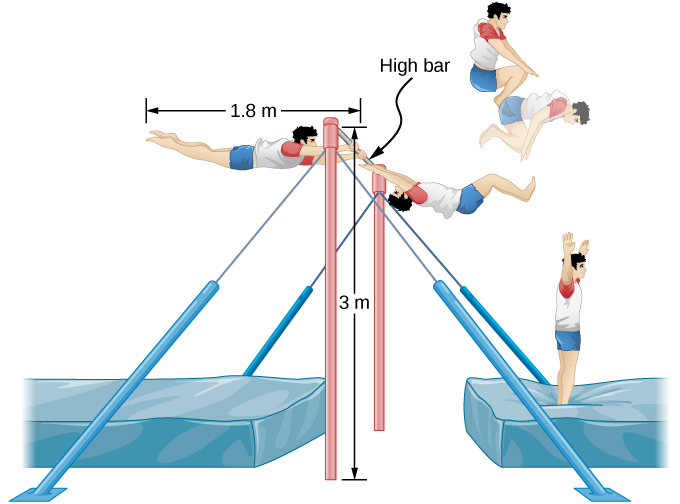Figure 11.17 A gymnast dismounts from a high bar and executes a number of revolutions in the tucked position before landing upright.

#### Strategy

Using conservation of angular momentum, we can find his rotation rate when in the tuck. Using the equations of kinematics, we can find the time interval from a height of 3.0 m to 1.8 m. Since he is moving horizontally with respect to the ground, the equations of free fall simplify. This will allow the number of revolutions that can be executed to be calculated. Since we are using a ratio, we can keep the units as rev/s and don’t need to convert to radians/s.

#### Solution

The moment of inertia at full extension is ${I}_{0}=\frac{1}{12}m{L}^{2}=\frac{1}{12}80.0\,\text{kg}(1{.8\,\text{m})}^{2}=21.6\,\text{kg}\cdot {\text{m}}^{2}$.

The moment of inertia in the tuck is ${I}_{\text{f}}=\frac{1}{12}m{L}_{\text{f}}^{\text{2}}=\frac{1}{12}80.0\,\text{kg}(0{.9\,\text{m})}^{2}=5.4\,\text{kg}\cdot {\text{m}}^{2}$.

Conservation of angular momentum: ${I}_{\text{f}}{\omega }_{\text{f}}={I}_{0}{\omega }_{0}\Rightarrow {\omega }_{\text{f}}=\frac{{I}_{0}{\omega }_{0}}{{I}_{\text{f}}}=\frac{21.6\,\text{kg}\cdot {\text{m}}^{2}(1.0\,\text{rev}\text{/}\text{s})}{5.4\,\text{kg}\cdot {\text{m}}^{2}}=4.0\,\text{rev}\text{/}\text{s}$.

Time interval in the tuck: $t=\sqrt{\frac{2h}{g}}=\sqrt{\frac{2(3.0-1.8)\text{m}}{9.8\,\text{m}\text{/}\text{s}}}=0.5\,\text{s}$.

In 0.5 s, he will be able to execute two revolutions at 4.0 rev/s.

#### Significance

Note that the number of revolutions he can complete will depend on how long he is in the air. In the problem, he is exiting the high bar horizontally to the ground. He could also exit at an angle with respect to the ground, giving him more or less time in the air depending on the angle, positive or negative, with respect to the ground. Gymnasts must take this into account when they are executing their dismounts.

### Example

#### Conservation of Angular Momentum of a Collision

A bullet of mass $m=2.0\,\text{g}$ is moving horizontally with a speed of $500.0\,\text{m}\text{/}\text{s}.$ The bullet strikes and becomes embedded in the edge of a solid disk of mass $M=3.2\,\text{kg}$ and radius $R=0.5\,\text{m}\text{.}$ The cylinder is free to rotate around its axis and is initially at rest (Figure). What is the angular velocity of the disk immediately after the bullet is embedded?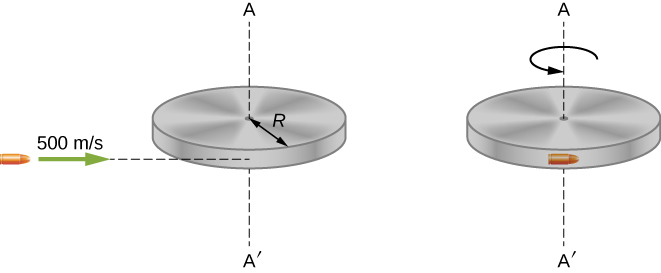Figure 11.18 A bullet is fired horizontally and becomes embedded in the edge of a disk that is free to rotate about its vertical axis.

#### Strategy

For the system of the bullet and the cylinder, no external torque acts along the vertical axis through the center of the disk. Thus, the angular momentum along this axis is conserved. The initial angular momentum of the bullet is $mvR$, which is taken about the rotational axis of the disk the moment before the collision. The initial angular momentum of the cylinder is zero. Thus, the net angular momentum of the system is $mvR$. Since angular momentum is conserved, the initial angular momentum of the system is equal to the angular momentum of the bullet embedded in the disk immediately after impact.

#### Solution

The initial angular momentum of the system is

${L}_{i}=mvR.$

The moment of inertia of the system with the bullet embedded in the disk is

$I=m{R}^{2}+\frac{1}{2}M{R}^{2}=(m+\frac{M}{2}){R}^{2}.$

The final angular momentum of the system is

${L}_{f}=I{\omega }_{f}.$

Thus, by conservation of angular momentum, ${L}_{i}={L}_{f}$ and

$mvR=(m+\frac{M}{2}){R}^{2}{\omega }_{f}.$

Solving for ${\omega }_{f},$

${\omega }_{f}=\frac{mvR}{(m+M\text{/}2){R}^{2}}=\frac{(2.0\times {10}^{-3}\,\text{kg})(500.0\,\text{m}\text{/}\text{s})}{(2.0\times {10}^{-3}\,\text{kg}\,+\,1.6\,\text{kg})(0.50\,\text{m})}=1.2\,\text{rad}\text{/}\text{s}.$

#### Significance

The system is composed of both a point particle and a rigid body. Care must be taken when formulating the angular momentum before and after the collision. Just before impact the angular momentum of the bullet is taken about the rotational axis of the disk.

### Summary

• In the absence of external torques, a system’s total angular momentum is conserved. This is the rotational counterpart to linear momentum being conserved when the external force on a system is zero.
• For a rigid body that changes its angular momentum in the absence of a net external torque, conservation of angular momentum gives ${I}_{f}{\omega }_{f}={I}_{i}{\omega }_{i}$. This equation says that the angular velocity is inversely proportional to the moment of inertia. Thus, if the moment of inertia decreases, the angular velocity must increase to conserve angular momentum.
• Systems containing both point particles and rigid bodies can be analyzed using conservation of angular momentum. The angular momentum of all bodies in the system must be taken about a common axis.

### Conceptual Questions

What is the purpose of the small propeller at the back of a helicopter that rotates in the plane perpendicular to the large propeller?

Show Solution

Without the small propeller, the body of the helicopter would rotate in the opposite sense to the large propeller in order to conserve angular momentum. The small propeller exerts a thrust at a distance R from the center of mass of the aircraft to prevent this from happening.

Suppose a child walks from the outer edge of a rotating merry-go-round to the inside. Does the angular velocity of the merry-go-round increase, decrease, or remain the same? Explain your answer. Assume the merry-go-round is spinning without friction.

As the rope of a tethered ball winds around a pole, what happens to the angular velocity of the ball?

Show Solution

The angular velocity increases because the moment of inertia is decreasing.

Suppose the polar ice sheets broke free and floated toward Earth’s equator without melting. What would happen to Earth’s angular velocity?

Explain why stars spin faster when they collapse.

More mass is concentrated near the rotational axis, which decreases the moment of inertia causing the star to increase its angular velocity.

Competitive divers pull their limbs in and curl up their bodies when they do flips. Just before entering the water, they fully extend their limbs to enter straight down (see below). Explain the effect of both actions on their angular velocities. Also explain the effect on their angular momentum.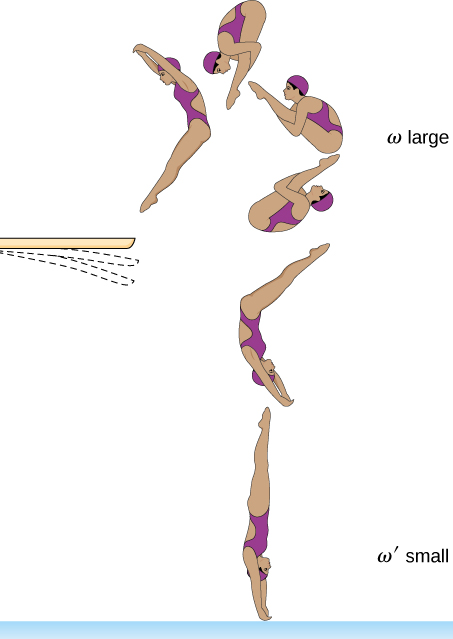### Problems

A disk of mass 2.0 kg and radius 60 cm with a small mass of 0.05 kg attached at the edge is rotating at 2.0 rev/s. The small mass suddenly separates from the disk. What is the disk’s final rotation rate?

The Sun’s mass is $2.0\times {10}^{30}\text{kg,}$ its radius is $7.0\times {10}^{5}\,\text{km,}$ and it has a rotational period of approximately 28 days. If the Sun should collapse into a white dwarf of radius $3.5\times {10}^{3}\,\text{km,}$ what would its period be if no mass were ejected and a sphere of uniform density can model the Sun both before and after?

Show Solution

${L}_{f}=\frac{2}{5}{M}_{S}{(3.5\times {10}^{3}\text{km})}^{2}\frac{2\pi }{{T}_{f}}$,

$\begin{array}{}\\ {(7.0\times {10}^{5}\,\text{km})}^{2}\frac{2\pi }{28\,\text{days}}={(3.5\times {10}^{3}\text{km})}^{2}\frac{2\pi }{{T}_{f}}\Rightarrow {T}_{f}\hfill \\ \\ \\ =28\,\text{days}\frac{{(3.5\times {10}^{3}\text{km})}^{2}}{{(7.0\times {10}^{5}\text{km})}^{2}}=7.0\times {10}^{-4}\text{day}=60.5\,\text{s}\end{array}$

A cylinder with rotational inertia ${I}_{1}=2.0\,\text{kg}\cdot {\text{m}}^{2}$ rotates clockwise about a vertical axis through its center with angular speed ${\omega }_{1}=5.0\,\text{rad}\text{/}\text{s}.$ A second cylinder with rotational inertia ${I}_{2}=1.0\,\text{kg}\cdot {\text{m}}^{2}$ rotates counterclockwise about the same axis with angular speed ${\omega }_{2}=8.0\,\text{rad}\text{/}\text{s}$. If the cylinders couple so they have the same rotational axis what is the angular speed of the combination? What percentage of the original kinetic energy is lost to friction?

A diver off the high board imparts an initial rotation with his body fully extended before going into a tuck and executing three back somersaults before hitting the water. If his moment of inertia before the tuck is $16.9\,\text{kg}\cdot {\text{m}}^{2}$ and after the tuck during the somersaults is $4.2\,\text{kg}\cdot {\text{m}}^{2}$, what rotation rate must he impart to his body directly off the board and before the tuck if he takes 1.4 s to execute the somersaults before hitting the water?

Show Solution

${f}_{\text{f}}=2.1\,\text{rev}\text{/}\text{s}\Rightarrow {f}_{0}=0.5\,\text{rev}\text{/}\text{s}$

An Earth satellite has its apogee at 2500 km above the surface of Earth and perigee at 500 km above the surface of Earth. At apogee its speed is 730 m/s. What is its speed at perigee? Earth’s radius is 6370 km (see below).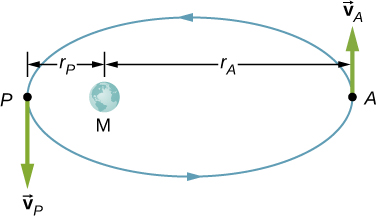A Molniya orbit is a highly eccentric orbit of a communication satellite so as to provide continuous communications coverage for Scandinavian countries and adjacent Russia. The orbit is positioned so that these countries have the satellite in view for extended periods in time (see below). If a satellite in such an orbit has an apogee at 40,000.0 km as measured from the center of Earth and a velocity of 3.0 km/s, what would be its velocity at perigee measured at 200.0 km altitude?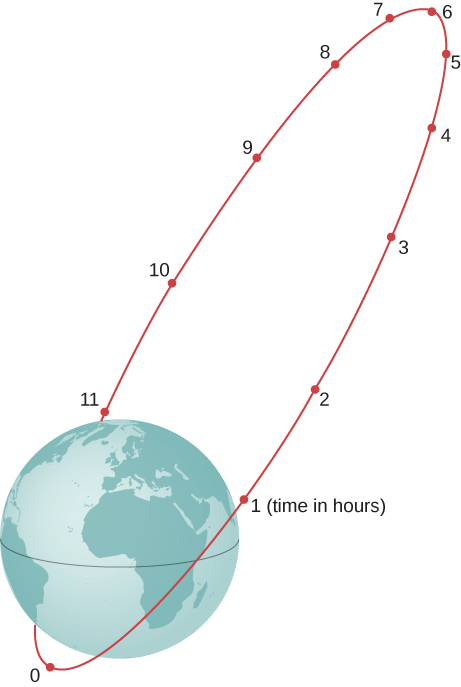${r}_{P}m{v}_{P}={r}_{A}m{v}_{A}\Rightarrow {v}_{P}=18.3\,\text{km}\text{/}\text{s}$

Shown below is a small particle of mass 20 g that is moving at a speed of 10.0 m/s when it collides and sticks to the edge of a uniform solid cylinder. The cylinder is free to rotate about its axis through its center and is perpendicular to the page. The cylinder has a mass of 0.5 kg and a radius of 10 cm, and is initially at rest. (a) What is the angular velocity of the system after the collision? (b) How much kinetic energy is lost in the collision?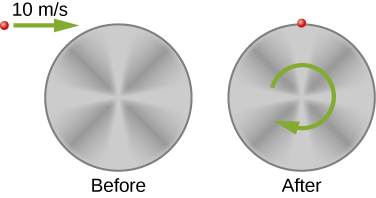A bug of mass 0.020 kg is at rest on the edge of a solid cylindrical disk $(M=0.10\,\text{kg,}\,R=0.10\,\text{m})$ rotating in a horizontal plane around the vertical axis through its center. The disk is rotating at 10.0 rad/s. The bug crawls to the center of the disk. (a) What is the new angular velocity of the disk? (b) What is the change in the kinetic energy of the system? (c) If the bug crawls back to the outer edge of the disk, what is the angular velocity of the disk then? (d) What is the new kinetic energy of the system? (e) What is the cause of the increase and decrease of kinetic energy?

Show Solution

a. ${I}_{\text{disk}}=5.0\times {10}^{-4}\,\text{kg}\cdot {\text{m}}^{2}$,

${I}_{\text{bug}}=2.0\times {10}^{-4}\,\text{kg}\cdot {\text{m}}^{2}$,

$\begin{array}{}\\ ({I}_{\text{disk}}+{I}_{\text{bug}}){\omega }_{1}={I}_{\text{disk}}{\omega }_{2},\hfill \\ \\ \\ {\omega }_{2}=14.0\,\text{rad}\text{/}\text{s}\hfill \end{array}$

b. $\Delta K=0.014\,\text{J}$;

c. ${\omega }_{3}=10.0\,\text{rad}\text{/}\text{s}$ back to the original value;

d. $\frac{1}{2}({I}_{\text{disk}}+{I}_{\text{bug}}){\omega }_{3}^{2}=0.035\,\text{J}$ back to the original value;

e. work of the bug crawling on the disk

A uniform rod of mass 200 g and length 100 cm is free to rotate in a horizontal plane around a fixed vertical axis through its center, perpendicular to its length. Two small beads, each of mass 20 g, are mounted in grooves along the rod. Initially, the two beads are held by catches on opposite sides of the rod’s center, 10 cm from the axis of rotation. With the beads in this position, the rod is rotating with an angular velocity of 10.0 rad/s. When the catches are released, the beads slide outward along the rod. (a) What is the rod’s angular velocity when the beads reach the ends of the rod? (b) What is the rod’s angular velocity if the beads fly off the rod?

A merry-go-round has a radius of 2.0 m and a moment of inertia $300\,\text{kg}\cdot {\text{m}}^{2}.$ A boy of mass 50 kg runs tangent to the rim at a speed of 4.0 m/s and jumps on. If the merry-go-round is initially at rest, what is the angular velocity after the boy jumps on?

Show Solution

${L}_{\text{i}}=400.0\,\text{kg}\cdot {\text{m}}^{2}\text{/}\text{s}$,

${L}_{\text{f}}=500.0\,\text{kg}\cdot {\text{m}}^{2}\omega$,

$\omega =0.80\,\text{rad}\text{/}\text{s}$

A playground merry-go-round has a mass of 120 kg and a radius of 1.80 m and it is rotating with an angular velocity of 0.500 rev/s. What is its angular velocity after a 22.0-kg child gets onto it by grabbing its outer edge? The child is initially at rest.

Three children are riding on the edge of a merry-go-round that is 100 kg, has a 1.60-m radius, and is spinning at 20.0 rpm. The children have masses of 22.0, 28.0, and 33.0 kg. If the child who has a mass of 28.0 kg moves to the center of the merry-go-round, what is the new angular velocity in rpm?

${I}_{0}=340.48\,\text{kg}\cdot {\text{m}}^{2}$, ${I}_{\text{f}}=268.8\,\text{kg}\cdot {\text{m}}^{2}$, ${\omega }_{\text{f}}=25.33\,\text{rpm}$

(a) Calculate the angular momentum of an ice skater spinning at 6.00 rev/s given his moment of inertia is $0.400\,\text{kg}\cdot {\text{m}}^{2}$. (b) He reduces his rate of spin (his angular velocity) by extending his arms and increasing his moment of inertia. Find the value of his moment of inertia if his angular velocity decreases to 1.25 rev/s. (c) Suppose instead he keeps his arms in and allows friction of the ice to slow him to 3.00 rev/s. What average torque was exerted if this takes 15.0 s?

Twin skaters approach one another as shown below and lock hands. (a) Calculate their final angular velocity, given each had an initial speed of 2.50 m/s relative to the ice. Each has a mass of 70.0 kg, and each has a center of mass located 0.800 m from their locked hands. You may approximate their moments of inertia to be that of point masses at this radius. (b) Compare the initial kinetic energy and final kinetic energy.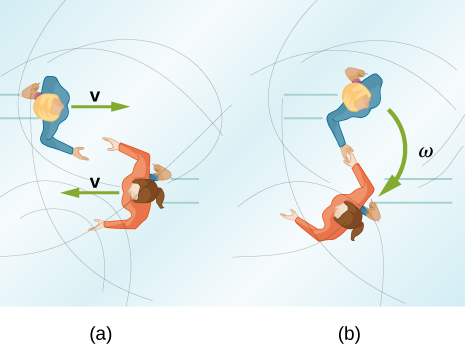a. $L=280\,\text{kg}\cdot {\text{m}}^{2}\text{/}\text{s}$, ${I}_{\text{f}}=89.6\,\text{kg}\cdot {\text{m}}^{2}$, ${\omega }_{\text{f}}=3.125\,\text{rad}\text{/}\text{s}$; b. ${K}_{\text{i}}=437.5\,\text{J}$, ${K}_{\text{f}}=437.5\,\text{J}$

A baseball catcher extends his arm straight up to catch a fast ball with a speed of 40 m/s. The baseball is 0.145 kg and the catcher’s arm length is 0.5 m and mass 4.0 kg. (a) What is the angular velocity of the arm immediately after catching the ball as measured from the arm socket? (b) What is the torque applied if the catcher stops the rotation of his arm 0.3 s after catching the ball?

In 2015, in Warsaw, Poland, Olivia Oliver of Nova Scotia broke the world record for being the fastest spinner on ice skates. She achieved a record 342 rev/min, beating the existing Guinness World Record by 34 rotations. If an ice skater extends her arms at that rotation rate, what would be her new rotation rate? Assume she can be approximated by a 45-kg rod that is 1.7 m tall with a radius of 15 cm in the record spin. With her arms stretched take the approximation of a rod of length 130 cm with $10%$ of her body mass aligned perpendicular to the spin axis. Neglect frictional forces.

Show Solution

Moment of inertia in the record spin: ${I}_{0}=0.5\,\text{kg}\cdot {\text{m}}^{2}$,

${I}_{\text{f}}=1.1\,\text{kg}\cdot {\text{m}}^{2}$,

${\omega }_{\text{f}}=\frac{{I}_{0}}{{I}_{\text{f}}}{\omega }_{0}\Rightarrow {f}_{\text{f}}=155.5\,\text{rev}\text{/}\text{min}$

A satellite in a geosynchronous circular orbit is 42,164.0 km from the center of Earth. A small asteroid collides with the satellite sending it into an elliptical orbit of apogee 45,000.0 km. What is the speed of the satellite at apogee? Assume its angular momentum is conserved.

A gymnast does cartwheels along the floor and then launches herself into the air and executes several flips in a tuck while she is airborne. If her moment of inertia when executing the cartwheels is $13.5\,\text{kg}\cdot {\text{m}}^{2}$ and her spin rate is 0.5 rev/s, how many revolutions does she do in the air if her moment of inertia in the tuck is $3.4\,\text{kg}\cdot {\text{m}}^{2}$ and she has 2.0 s to do the flips in the air?

Show Solution

Her spin rate in the air is: ${f}_{\text{f}}=2.0\,\text{rev}\text{/}\text{s}$;

She can do four flips in the air.

The centrifuge at NASA Ames Research Center has a radius of 8.8 m and can produce forces on its payload of 20 gs or 20 times the force of gravity on Earth. (a) What is the angular momentum of a 20-kg payload that experiences 10 gs in the centrifuge? (b) If the driver motor was turned off in (a) and the payload lost 10 kg, what would be its new spin rate, taking into account there are no frictional forces present?

A ride at a carnival has four spokes to which pods are attached that can hold two people. The spokes are each 15 m long and are attached to a central axis. Each spoke has mass 200.0 kg, and the pods each have mass 100.0 kg. If the ride spins at 0.2 rev/s with each pod containing two 50.0-kg children, what is the new spin rate if all the children jump off the ride?

Show Solution

Moment of inertia with all children aboard:

${I}_{0}=2.4\times {10}^{5}\,\text{kg}\cdot {\text{m}}^{2}$;

${I}_{\text{f}}=1.5\times {10}^{5}\,\text{kg}\cdot {\text{m}}^{2}$;

${f}_{\text{f}}=0.3\text{rev}\text{/}\text{s}$

An ice skater is preparing for a jump with turns and has his arms extended. His moment of inertia is $1.8\,\text{kg}\cdot {\text{m}}^{2}$ while his arms are extended, and he is spinning at 0.5 rev/s. If he launches himself into the air at 9.0 m/s at an angle of $45^\circ$ with respect to the ice, how many revolutions can he execute while airborne if his moment of inertia in the air is $0.5\,\text{kg}\cdot {\text{m}}^{2}$?

A space station consists of a giant rotating hollow cylinder of mass ${10}^{6}\,\text{kg}$ including people on the station and a radius of 100.00 m. It is rotating in space at 3.30 rev/min in order to produce artificial gravity. If 100 people of an average mass of 65.00 kg spacewalk to an awaiting spaceship, what is the new rotation rate when all the people are off the station?

Show Solution

${I}_{0}=1.00\times {10}^{10}\,\text{kg}\cdot {\text{m}}^{2}$,

${I}_{\text{f}}=9.94\times {10}^{9}\,\text{kg}\cdot {\text{m}}^{2}$,

${f}_{\text{f}}=3.32\,\text{rev}\text{/}\text{min}$

Neptune has a mass of $1.0\times {10}^{26}\,\text{kg}$ and is $4.5\times {10}^{9}\text{km}$ from the Sun with an orbital period of 165 years. Planetesimals in the outer primordial solar system 4.5 billion years ago coalesced into Neptune over hundreds of millions of years. If the primordial disk that evolved into our present day solar system had a radius of ${10}^{11}$ km and if the matter that made up these planetesimals that later became Neptune was spread out evenly on the edges of it, what was the orbital period of the outer edges of the primordial disk?

### Glossary

law of conservation of angular momentum
angular momentum is conserved, that is, the initial angular momentum is equal to the final angular momentum when no external torque is applied to the system